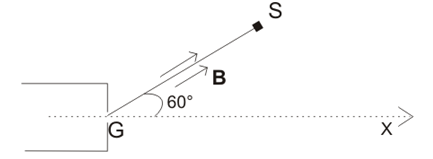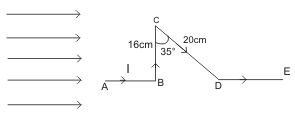# Important questions on Magnetic field and magnetic effects of current for JEE Main And Advanced

Question 1- A current of 10A is flowing from east to west in a long wire kept in east-west direction. Find the magnetic field in a horizontal plane at a distance of (1) 10cm north (2) 20cm south from the wire; and in a vertical plane at a distance of (3) 30cm downward (4) 50cm upward.
Question 2- A short conductor of length 4cm is placed parallel to the long conductor of length 2m near to its centre at a distance of 2cm. The conductor carry currents of 2A and 5A respectively in opposite directions. Find the total force exert on the long conductor.
Question 3- An electron-gun G emit electron of energy 2KeV travelling in the positive X-direction. The electron are required to hit the spot S where GS= 0.1m, and the line GS makes an angle of 600 with X-axis, as shown in the figure. A uniform magnetic field B parallel to GS exist in the region outside the electronic-gun. Find the minimum value of B needed to make the electron hit S.
Given: me =9.1 x 10-31 kg , e=1.6 x 10-19 CQuestion 4- A particle of mass 1x 10-26   kg  and charge 1.6 x 10-19  C travelling with velocity of 1.28 x 106  m/s  in +x direction enters a region having electric field E and a uniform magnetic field B such as Ex =Ey =0, Ez = -102.4 V/m and Bx =Bz =0, By =8x10-2 Wb/m . The particle enters this region at a long time t=0. Determine the location (x, y and z co-ordinates) of the particle at t=5x10-6 s . If the electric field switched off at this instant (with the magnetic field still present), what will be the position of the particle at t=7.46 x10-6 s.

Question 5- In a certain region surrounding the origin of the coordinates, B=5 x10-4  az T  and E=5az V/m. A proton ( qp =1.602 x 10-19  C,mp  =1.6 x10-27  kg) enters in the field at the origin with a velocity u0 =2.5 x105  ax m/s. Describe the proton’s motion and give its position after three complete revolutions
Question 6- Find an expression for magnetic field at the centre of a circular current carrying loop.
Question 7- A current of 1.0 A is flowing in the sides of an equilateral triangle of sides 4.5 x 10-2m. Find the magnetic field at the centroid of the triangle.
Question 8- A charge q=40 µC  moves with instantaneous velocity u=(5x104m/s through the uniform fields   E=(6x104) (.52 i  +.56j +.645 k) V/m , B=(1.7)(.693 i + .6 j + .4k) T find the magnitude and direction of the instantaneous force on q.
Question 9- Find the force on each segment of the wire as shown below in the figureif B=0.15T. Assume that the current in the wire is 5A.

Question 10- A planar coil of 12 turns carries 15A. The coil is oriented with respect to the uniform magnetic field B=0.2i+0.3j-0.4k T such that its directed area is A=0.04i-0.05j+0.07k m2. Find (a) the dipole moment of the coil (b)the potential energy of the in the given orientation, and (c)the angle between the positive normal to the coil and the field.

Solutions

Note to our visitors :-

Thanks for visiting our website.
DISCLOSURE: THIS PAGE MAY CONTAIN AFFILIATE LINKS, MEANING I GET A COMMISSION IF YOU DECIDE TO MAKE A PURCHASE THROUGH MY LINKS, AT NO COST TO YOU. PLEASE READ MY DISCLOSURE FOR MORE INFO.